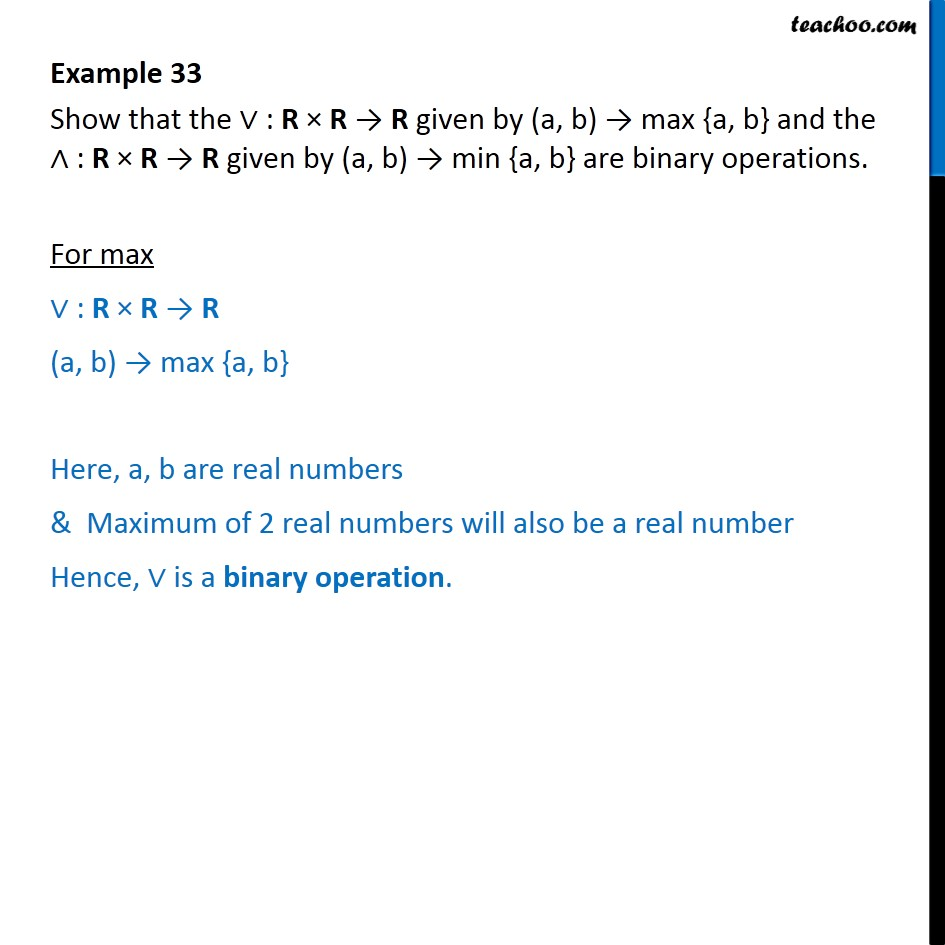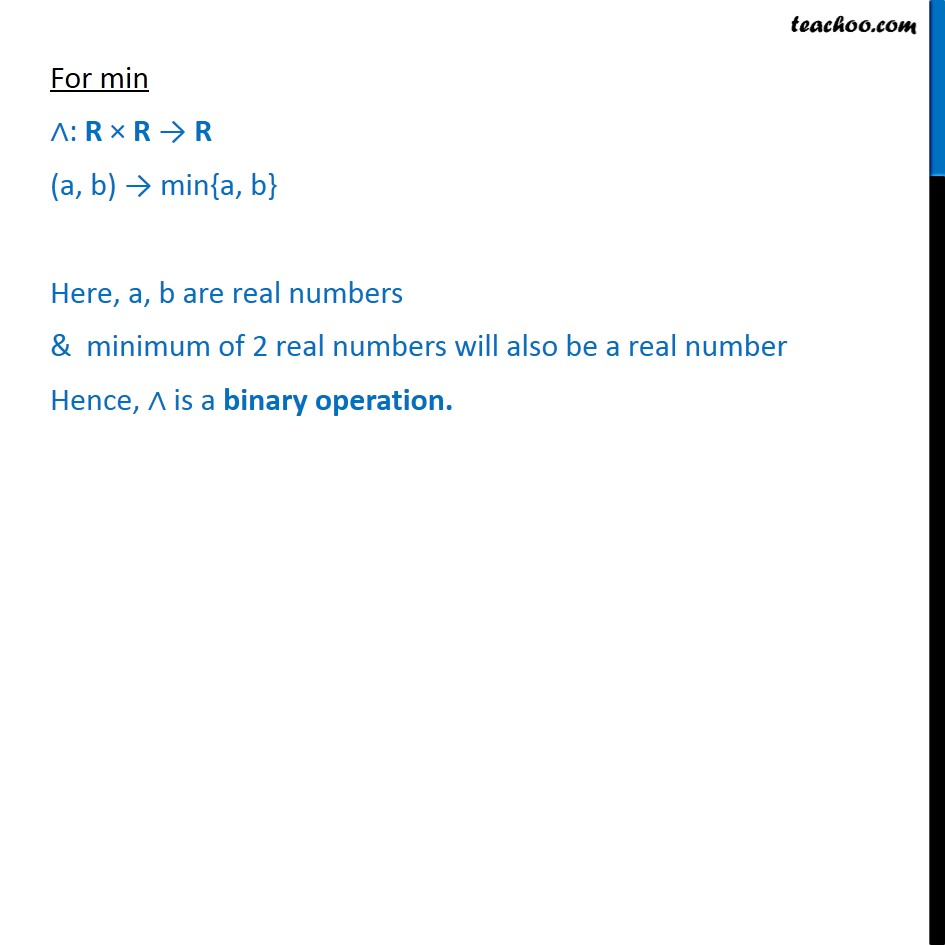Examples

Chapter 1 Class 12 Relation and Functions
Serial order wiseGet live Maths 1-on-1 Classs - Class 6 to 12

### Transcript

Example 33 Show that the : R R R given by (a, b) max {a, b} and the : R R R given by (a, b) min {a, b} are binary operations. For max : R R R (a, b) max {a, b} Here, a, b are real numbers & Maximum of 2 real numbers will also be a real number Hence, is a binary operation. For min : R R R (a, b) min{a, b} Here, a, b are real numbers & minimum of 2 real numbers will also be a real number Hence, is a binary operation.